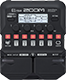# NEWAVE

Discussion in 'Zoom G1/G1X Four' started by Ruling, Jun 5, 2022.

1. NEWAVEDevice: Zoom G1 Four
Firmware: 2.00

Name on device: NEWAVE
Optimized for: Phones/Speaker

Effects chain:You may find this patch useful for a kind of synth lead

Effect: "ZNR" (Dynamics), active - "yes"
"Detect" = EFXIN
"Depth" = 100
"Threshold" = 65
"Decay" = 21

Effect: "NYC Muff" (Overdrive / Distortion), active - "yes"
"Sustain" = 100
"Tone" = 55
"Balance" = 100
"Volume" = 70

Effect: "Gt GEQ" (Filter), active - "yes"
"160" = 0.0
"400" = 0.0
"800" = 0.0
"3.2k" = 0.0
"6.4k" = 0.0
"12k" = 0.0
"VOL" = 80

Effect: "SuperCho" (Modulation), active - "yes"
"Depth" = 70
"Rate" = 50
"Tone" = 50
"Mix" = 60

Effect: "Dual DLY" (Delay), active - "yes"
"TimeA" = 500
"F.B A" = 50
"TimeB" = 375
"F.B B" = 50
"DlyMx" = 25
"Balance" = 40
"Depth" = MN-0
"Speed" = 25

Patch Volume: 60